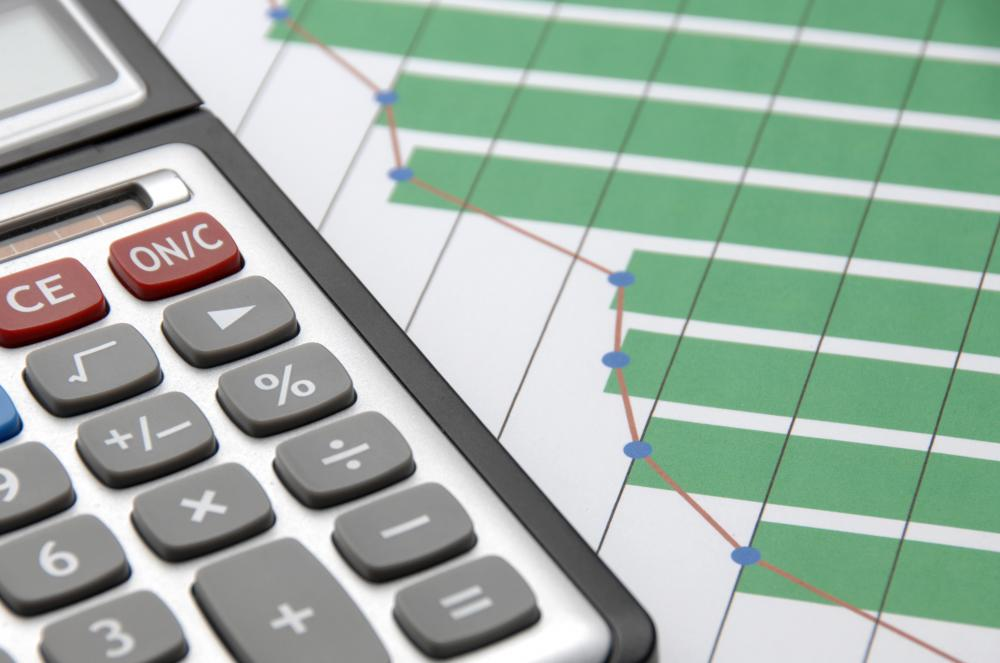# What Is Involved in Calculating CPI?

Osmand Vitez

The consumer price index (CPI) is a standard calculation for determining inflation in a nation’s economy. It is one of the most commonly calculated and reported economic measures economists use to judge the health and strength of the economy. Calculating CPI has two different formulas, one for a single item and one for a group of items. The monthly CPI figure reported through news outlets is generally the latter formula, which represents a basket of goods most often purchased by all consumers. Each formula divides the current price for each item by a base year and multiplies the result by 100 to get a percentage of inflation; calculating CPI for multiple goods may involve weighting each item in the basket of goods.The monthly CPI figure reported through news outlets generally represents a basket of goods most often purchased by all consumers.

Economists often select a base year in which to start CPI calculations. This year is not always important, though it must remain the same for each year thereafter for the formula to make sense. For example, economists may agree that 1984 is a good starting point for calculating CPI. The base year price for each good in the CPI formula, therefore, will use each good’s price from this year. Adjustments or changes may be necessary to take inflation measurements over a longer period of time.Economists calculate the rate of price increases by comparing current costs for goods to those in an established base year.

Calculating CPI for a single item is fairly simple. Economists simply divide the current year’s price by the base period price for the item and then multiply the result by 100. The result is a percentage of inflation that economists claim increased the price of that item. For example, a 2.1 percent increase in the price of a gallon of milk is a common answer for a single CPI calculation. The biggest problem, however, is that the calculation does not necessarily indicate why the price increased.

The fictional basket of goods in calculating CPI is a bit more involved. Economists must select a group of items — such as food, housing, clothes, vehicles, medical care, and other goods — that accurately represent the needs for an individual to sustain a standard of living. Weighting each item can be difficult; for example, economists may weight housing by 40 percent, food by 18 percent, and medical care by nine percent, with the other items making up the remainder. Calculating CPI is the same as the previous formula for each individual item, with the answer multiplied by the weight factor for the basket of goods. Adding together the final figures presents a total inflation calculation that should represent the amount of inflation in the current market.

## You might also Like